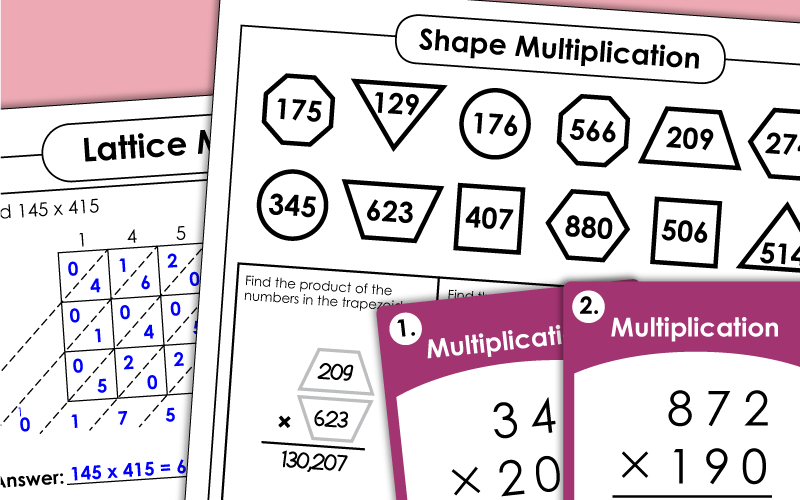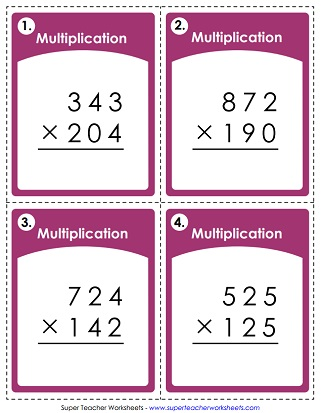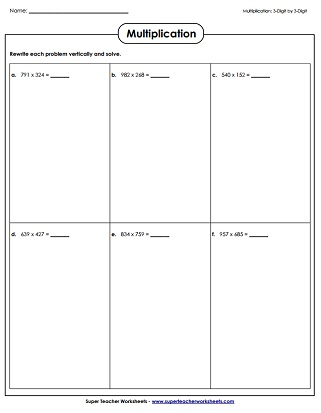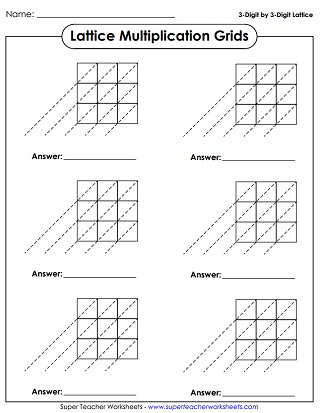# Multiplication by 3-Digit Numbers

With these multiplication worksheets, student can practice multiplying by 3-digit numbers. (example: 491 x 612)## Multiplying by 3-Digit Numbers

Graph Paper Math Drills; 3-digits times 3-digits (example: 667 x 129)
Each problem on this worksheet is written horizontally. Students rewrite the problems vertically and solve. (example: 334 x 109)
Find the products of the numbers in the specified shapes. For example: "Find the product of the numbers in the hexagons."
This file has 30 task cards with vertical 3-digit by 3-digit multiplication problems.

Multiplication Worksheet Generator Tool

Make your own customized multiplication worksheets with this tool. You simply choose the number of digits you'd like in the first and second factor. Tell if you'd like the problems horizontal or vertical. There are also options to include word problems.

## Lattice Multiplication

For this activity, students will use a lattice grid to calculate the products of numbers. Includes an explanation and many practice grids.
This page has six blank lattice grids. Each one is designed for multiplying pairs of triple-digit numbers.

Basic Multiplication Worksheets

## Sample Worksheet ImagesMy Account
Site Information